Vizard 7 » Reference » Shaders » Post-Process Effects » Distort effects

## Distort effects

The vizfx.postprocess.distort library is a collection of effects that perform various distortions on the rendered image by modifying the texture coordinates of each pixel.

#### Pincushion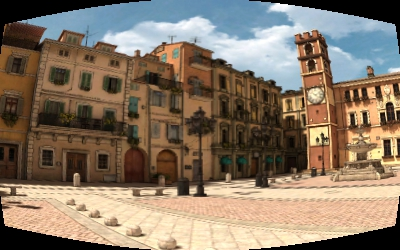Applies a pincushion/barrel distortion on the image.

 Constructor Description PincushionEffect(     k1 = 0.0,     scaleToFit = True ) Create the pincushion effect with the initial k1 factor and scaleToFit setting.

 Method Description .setK1(value) Set the k1 value of the distortion. A positive value results in a barrel distortion, a negative value results in a pincushion distortion. .getK1() Get the k1 distortion value. .setScaleToFit(value) Set whether distortion is scaled to fit resolution of output image. .getScaleToFit() Get scale to fit setting.

This effect can be registered with vizconfig.

import vizfx.postprocess
from vizfx.postprocess.distort import PincushionEffect
effect = PincushionEffect(0.5)

#### Pixelate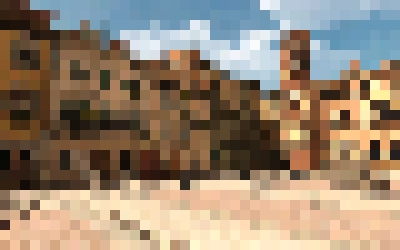Pixelates the image.

 Constructor Description PixelateEffect(pixelSize = (1,1)) Create the pixelate effect with the initial pixelSize.

 Method Description .setPixelSize(size) Set the [width,height] pixel size. This is the number of actual pixels that will make up the virtual pixel. .getPixelSize() Get the [width,height] pixel size.

This effect can be registered with vizconfig.

import vizfx.postprocess
from vizfx.postprocess.distort import PixelateEffect
effect = PixelateEffect([10,10])

#### Bulge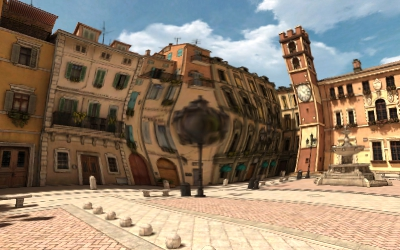Bulges the image.

 Constructor Description BulgeEffect(    bulge = 0.0,    radius = 0.5,    center = (0.5,0.5)) Create the bulge effect with the initial bulge factor, radius, and center.

 Method Description .setBulge(value) Set the bulge factor. Typically ranges from -1 to 1. Positive value bulges the image outwards, negative value bulges the image inward. .getBulge() Get the bulge factor. .setRadius(radius) Set the bulge radius in normalized (0-1) image units. .getRadius() Get the bulge radius in normalized (0-1) image units. .setCenter(center) Set the bulge [x,y] center in normalized (0-1) image units. .getCenter() Get the bulge [x,y] center in normalized (0-1) image units.

This effect can be registered with vizconfig.

import vizfx.postprocess
from vizfx.postprocess.distort import BulgeEffect
effect = BulgeEffect(0.5)

#### Twist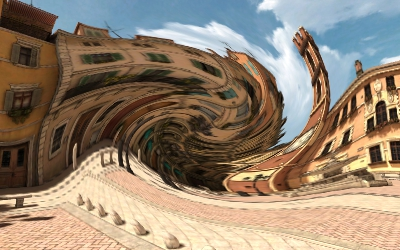Twists the image.

 Constructor Description TwistEffect(    twist = 0.0,    radius = 0.5,    center = (0.5,0.5)) Create the twist effect with the initial twist angle, radius, and center.

 Method Description .setTwist(twist) Set the twist angle in degrees. Positive value twists the image clockwise, negative value twists the image counter-clockwise. .getTwist() Get the twist angle in degrees. .setRadius(radius) Set the twist radius in normalized (0-1) image units. .getRadius() Get the twist radius in normalized (0-1) image units. .setCenter(center) Set the twist [x,y] center in normalized (0-1) image units. .getCenter() Get the twist [x,y] center in normalized (0-1) image units.

This effect can be registered with vizconfig.

import vizfx.postprocess
from vizfx.postprocess.distort import TwistEffect
effect = TwistEffect(180)

#### Wave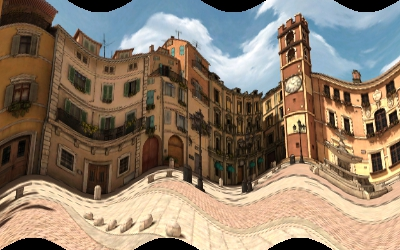Applies a sine wave to the image.

 Constructor Description WaveEffect(    amplitude = 0.0,    period = 1.0,    offset = 0.0,    angle = 0.0,    repeat = False) Create the wave effect with the initial parameters.

 Method Description .setAmplitude(twist) Set the amplitude of the wave in normalized (0-1) image units. .getAmplitude() Get the amplitude of the wave in normalized (0-1) image units. .setPeriod(radius) Set the period of the wave in normalized (0-1) image units. .getPeriod() Get the period of the wave in normalized (0-1) image units. .setOffset(center) Set the offset of the wave along the horizontal axis in normalized (0-1) image units. .getOffset() Get the offset of the wave along the horizontal axis in normalized (0-1) image units. .setAngle(value) Set the angle of the vertical axis of the wave, in degrees. 0 is upward, and positive angles go clockwise. .getAngle() Get the angle of the vertical axis of the wave, in degrees. .setRepeat(value) Set whether to repeat the image when UV coordinates are outside (0-1) range. .getRepeat() Get whether to repeat the image when UV coordinates are outside (0-1) range.

This effect can be registered with vizconfig.

import vizfx.postprocess
from vizfx.postprocess.distort import WaveEffect
effect = WaveEffect(amplitude=0.05, period=3.0)